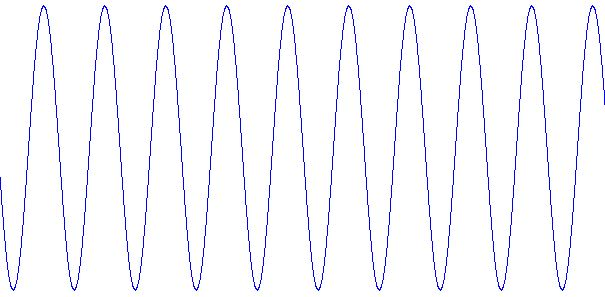# Java swing program to print a wave form with output

In this java swing program, we are going to learn how to make a wave form and print it on the output screen? Here, we are using classes and methods of 'java.awt' and 'javax.swing' packages.
Submitted by IncludeHelp, on November 03, 2017

This is an example of Java swing; here we are going to print a wave form. To implement this program, we are using two packages and its classes, objects: java.awt.* and javax.swing.*

### Program to print a wave form using in java

```import java.awt.*;
import javax.swing.*;

public class WavePrint extends JPanel
{
int SCALEFACTOR = 200;
int cycles;
int points;
double[] sines;
int[] pts;

public  void setCycles(int cycles)
{
this.cycles = cycles;
this.points = SCALEFACTOR * cycles * 2;
this.sines = new double[points];
for (int i = 0; i < points; i++)
{
double radians = (Math.PI / SCALEFACTOR) * i;
}
}

public void paintComponent(Graphics g)
{
int maxWidth = getWidth();
double hstep = (double) maxWidth / (double) points;
int maxHeight = getHeight();
pts = new int[points];
for (int i = 0; i < points; i++)
{
pts[i] = (int) (sines[i] * maxHeight / 2 * .95 + maxHeight / 2);
}
g.setColor(Color.BLUE);
for (int i = 1; i < points; i++)
{
int x1 = (int) ((i - 1) * hstep);
int x2 = (int) (i * hstep);
int y1 = pts[i - 1];
int y2 = pts[i];
g.drawLine(x1, y1, x2, y2);
}
}

public static void main(String[] args)
{
JFrame.setDefaultLookAndFeelDecorated(true);
JFrame frame = new JFrame("Print the wave pattern");
frame.setDefaultCloseOperation(JFrame.EXIT_ON_CLOSE);
frame.setBackground(Color.white);
frame.setSize(500, 200);

WavePrint sw = new WavePrint();
sw.setCycles(10);
frame.setVisible(true);
}
}
```

OutputTop MCQs

Languages: » C » C++ » C++ STL » Java » Data Structure » C#.Net » Android » Kotlin » SQL
Web Technologies: » PHP » Python » JavaScript » CSS » Ajax » Node.js » Web programming/HTML
Solved programs: » C » C++ » DS » Java » C#
Aptitude que. & ans.: » C » C++ » Java » DBMS
Interview que. & ans.: » C » Embedded C » Java » SEO » HR
CS Subjects: » CS Basics » O.S. » Networks » DBMS » Embedded Systems » Cloud Computing
» Machine learning » CS Organizations » Linux » DOS
More: » Articles » Puzzles » News/Updates